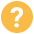Operations research problem coder needed

Payment method: Verified

Evaluating bids
Description:
We have an operations research problem.
Processing time of the script to finish task must be minimal (less than 60seconds). Therefore we should choose best possible algorithm at beginning for our problem.

Please consider: dynamic programming, knap sack problem, eager algorithm, compression, constraint satisfactory programming.

We have some random data in a csv file with following fields:
amount, pos1, pos2, pos3, pos4.
One row in csv file is considered a "line".
Multiple lines must be placed on a invoice.

Csv data will be provided.

We have two problems to solve with a script:
1) select pos1, pos2, pos3 and pos4 out of all data lines in such a combination that you maximize the number of lines you write on one invoice, where you at same time minimize the number of wrong lines.

2) all lines have an amount. You have to group all lines to amount segments, following are possible: 0.12\$, 0.24\$, 0.48\$, 0.84\$, 0.96\$, 1.2\$, 1.8\$, 2.4\$, 3\$, 4\$, 5\$, 6\$, 7\$, 8\$, 9\$, 10\$
one one invoice only lines with same amount group can be set.

And following restrictions:
maximal number of invoices we can have is 100
+ initial source data (csv) has 4000 to 6000 lines (it varies from day to day).

=> Outcome expected: a script (can be c++, python, php, go, whatever…) which writes out:
invoice #1:  4 lines a 4\$ with pos1=1, pos2=1, pos3=1,pos4=2 +  pos1=1, pos2=1, pos3=1,pos4=3 +  pos1=1, pos2=1, pos3=1,pos4=4 +  pos1=1, pos2=1, pos3=1,pos4=5
invoice #2:  2 lines a 0.24\$ with pos1=1, pos2=2, pos3=2,pos4=1 +  pos1=1, pos2=2, pos3=3,pos4=1

Category: IT & Programming
Subcategory: Web development
What is the scope of the project?: Small change or bug
Is this a project or a position?: Project
I currently have: I have specifications
Required availability: As needed

USD 100 - 250Budget

Bids

8

Interested freelancers

Published: Last month